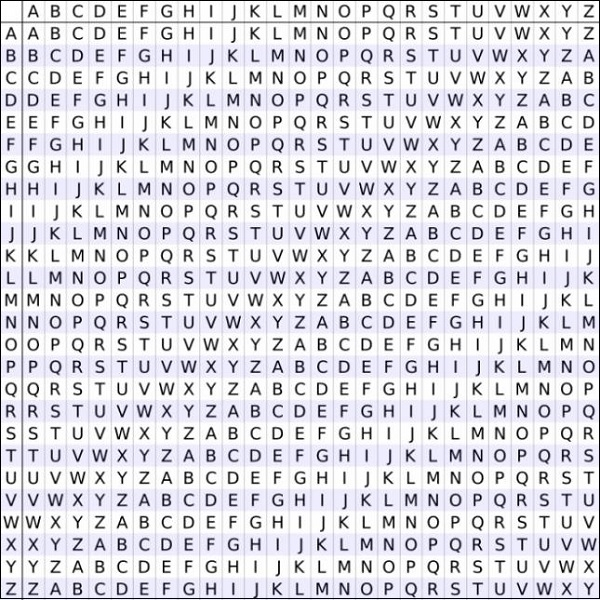# C++ Program to Implement the Vigenere Cypher

Vigenere Cipher is a kind of polyalphabetic substitution method of encrypting alphabetic text.

Vigenere Cipher Table is used in which alphabets from A to Z are written in 26 rows, for encryption and decryption in this method.## Encryption

Key: WELCOME

Message: Thisistutorialspoint

Here we have to obtain a key by repeating the given key till its length becomes equal to original message length.

For encryption take first letter of message and key i.e. T and W. Take the alphabet in Vigenere Cipher Table where T row and W column coincides i.e. P.

Repeat the same process for all remaining alphabets in message text.

Finally, the encrypted message text is −

Encrypted Message: PLTUWEXQXZTWMPOTZKBF.

The cipher text can be generated by below equation.

Ei = (Pi + Ki) mod 26

Here P is plain text and K is key.

## Decryption

Key: WELCOME

Encrypted Message: PLTUWEXQXZTWMPOTZKBF

Take generated key and first alphabet of encrypted message i.e. P and W. Analyze Vigenere Cipher Table, look for alphabet P in column W, the corresponding row will be the first alphabet of original message i.e. T.

Repeat this process for all the alphabets in encrypted message.

Original Message: Thisistutorialspoint

This can be represented in algebraic form by following equation.

Pi = (Ei – Ki + 26) mod 26

Here is a C++ program to implement Vigenere cipher.

## Algorithms

Begin
Function encryption(string t)
for i = 0, j = 0 to t.length() - 1
char c = t[i]
if (c >= 'a' and c <= 'z')
c = c + 'A' - 'a'
else if (c < 'A' or c > 'Z')
continue
output = output + (c + k[j] ) % 26 + 'A'
j = (j + 1) % k.length()
return output
End
Begin
Function decryption(string t)
for i = 0, j = 0 to t.length() - 1
char c = t[i]
if (c >= 'a' and c <= 'z')
c = c + 'A' - 'a'
else if (c < 'A' or c > 'Z')
continue
output =output + (c - k[j] + 26) % 26 + 'A'
j = (j + 1) % k.length()
return output
End

## Example

#include <iostream>
#include <string>
using namespace std;
class Vig {
public:
string k;
Vig(string k) {
for (int i = 0; i < k.size(); ++i) {
if (k[i] >= 'A' && k[i] <= 'Z')
this->k += k[i];
else if (k[i] >= 'a' && k[i] <= 'z')
this->k += k[i] + 'A' - 'a';
}
}
string encryption(string t) {
string output;
for (int i = 0, j = 0; i < t.length(); ++i) {
char c = t[i];
if (c >= 'a' && c <= 'z')
c += 'A' - 'a';
else if (c < 'A' || c > 'Z')
continue;
output += (c + k[j] - 2 * 'A') % 26 + 'A'; //added 'A' to bring it in range of ASCII alphabet [ 65-90 | A-Z ]
j = (j + 1) % k.length();
}
return output;
}
string decryption(string t) {
string output;
for (int i = 0, j = 0; i < t.length(); ++i) {
char c = t[i];
if (c >= 'a' && c <= 'z')
c += 'A' - 'a';
else if (c < 'A' || c > 'Z')
continue;
output += (c - k[j] + 26) % 26 + 'A';//added 'A' to bring it in range of ASCII alphabet [ 65-90 | A-Z ]
j = (j + 1) % k.length();
}
return output;
}
};
int main() {
Vig v("WELCOME");
string ori ="Thisistutorialspoint";
string encrypt = v.encryption(ori);
string decrypt = v.decryption(encrypt);
cout << "Original Message: "<<ori<< endl;
cout << "Encrypted Message: " << encrypt << endl;
cout << "Decrypted Message: " << decrypt << endl;
}

## Output

Original Message: Thisistutorialspoint
Encrypted Message: PLTUWEXQXZTWMPOTZKBF
Decrypted Message: THISISTUTORIALSPOINT

Updated on: 30-Jul-2019

4K+ Views